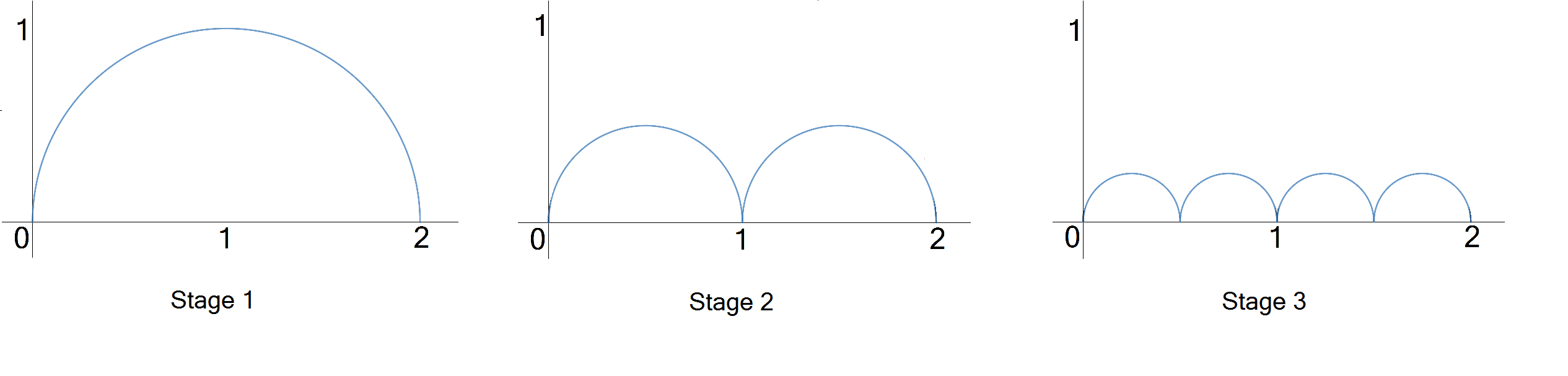Geometry

# Deep Dive: Pointwise and Uniform Convergence 1A sequence of functions $f_n(x)$ is drawn in stages. Each stage in the sequence consists of a connected path of semicircles:

$f_1(x)$ consists of 1 semicircle of radius 1,

$f_2(x)$consists of 2 semicircles of radius $\frac{1}{2},$

$f_3(x)$consists of 4 semicircles of radius $\frac{1}{4},$

and so on.

As $n$ increases and this process continues indefinitely, what happens to the lengths of $f_n(x)$ over the interval $[0,2]?$True or False?

Let $f_n(x)$ be the function on $[0,2]$ whose graph is the Stage $n$ semicircles. Then $f_n\left(\frac{1}{3}\right) \rightarrow 0,$ i.e.,

$\lim_{n \to \infty} f_n\left(\frac{1}{3}\right) = 0.$True or False?

Let $f_n(x)$ be the function on $[0,2]$ whose graph is the Stage $n$ semicircles. Then for all $x$ in $[0,2],$ $f_n\left(x\right) \rightarrow 0,$ i.e.,

$\lim_{n \to \infty} f_n\left(x\right) = 0.$

Note. Another way of phrasing the question is: "Does the sequence $\{f_n(x)\}$ converge pointwise to $f(x) = 0$ on the interval $[0,2]?$True or False?

Let $f_n(x)$ be the function on $[0,2]$ whose graph is the Stage $n$ semicircles. Then for all $x$ in $[0,2]$ where they are defined, the derivatives $(f_n)'\left(x\right) \rightarrow 0,$ i.e.,

$\lim_{n \to \infty} (f_n)'\left(x\right) = 0.$

Note. 0 is the derivative of the function $f(x) = 0$ that the functions $f_n$ approach.

True or False?

Suppose $\{f_n\}$ and $f$ are functions on an interval $I,$ and $L(g)$ represents the length of the curve $g$ on $I.$

If $f_n(x) \to f(x)$ for all $x \in I,$ then $L(f_n) \to L(f).$

Alternate phrasings:

If $\lim_{n \to \infty} f_n(x) = f(x)$ for all $x \in I,$ then $\lim_{n \to \infty}L(f_n) = L(f).$

If the sequence of curves approach $f$ at each point, then the lengths of the curves approach the length of $f.$

×